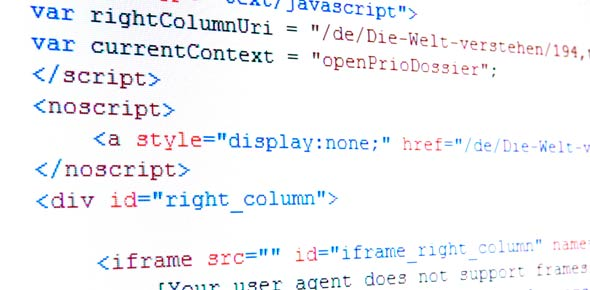# Programming Algorithms

20 Questions | Total Attempts: 1224SettingsThis quiz test the knowledge of SJC students based on the introduction to programming, the definition of algorithms and they different formats of pseudocode and flowcharts. Created by Ms. Stacy Sutherland

• 1.
What is a sequence of precise instructions for solving a problem in a given amount of time.
• A.

Pseudocode

• B.

Program

• C.

Algorithm

• D.

Flowchart

• 2.
Algorithms must be logical,  precise, unambiguous, correct and _____________
• 3.
Which is the algorithmic structure in proper order:
• A.

• B.

• C.

• D.

• 4.
The three types of control structures that an algorithm can have are:
• 5.
Input, Output, Arithmetic operation and variable assignment statements are examples of which type of structure:
• A.

Loop

• B.

Sequential

• C.

Array

• D.

Selection

• E.

Repetition

• 6.
Maximum=20 and count=1 are examples of statements that:
• A.

Assign variables

• B.

Arithmetic operations

• C.

Output

• D.

Input

• 7.
Print total-cost and display average are examples of statements that:
• A.

Assign variables

• B.

Involves arithmetic operations

• C.

Input

• D.

Output

• 8.
Words that involves input are all of the following except:
• A.

Get

• B.

Print

• C.

Accept

• D.

• 9.
Which of the following words wouldn't be used in a selection structure:
• A.

Else

• B.

Then

• C.

If

• D.

Repeat

• 10.
What two forms can constitute pseudocode?
• 11.
A flowchart is a ______________ representation of an algorithm.
• 12.
In a flowchart the parallelogram is used to:
• A.

Processing

• B.

Decisions

• C.

Input and output

• D.

Start and stop terminators

• 13.
In a flowchart the diamond is used for:
• A.

Start and stop terminators

• B.

Input and output

• C.

Decisions

• D.

Processing

• 14.
In a flowchart terminators for start and stop are usually indicated by what shape?
• A.

Square

• B.

Oval

• C.

Rhombus

• D.

Circle

• 15.
____________ structure allow statements to be repeated a fixed number of times or until some condition evaluated to false.
• 16.
In a loop if the exact number of repetitions is unknown beforehand and is based on some condition, then it is called ____________ loop.
• 17.
In a flowchart what symbol often shows the flow of logic?
• 18.
In a loop if the number of repetitions is known beforehand, then the loop structure is called a _______________ loop.
• 19.
Give the name of the programming language that we are going to use for IT CSEC?
• 20.
Pascal is a high level language, from which generation did this language came from?
Related TopicsBack to top